## Using XY-pic in LyX

January 26, 2006

Abstract

With the current version of LY X and with the preview-style installed in the LATEX-System, the graph drawing package XY -Pic can now be conveniently used inside LY X. Diagrams can be edited and displayed inside the main LY X editing window. Here, we shall describe how to use the \xymatrix command from xypic inside LY X in order to draw, to edit and to preview diagrams as typically used in category theory, algebra, and related fields.

### Contents

3.2 Arrows
3.3 Labels

3.4.1 Arrow design

### 1 Introduction

The xypic-package has long served as a convenient tool for easily constructing graphs and diagrams in LATEX. Unfortunately, its use in LY X had long been restricted to the infamous ERT-boxes, meaning that the LY X editor could only display the LATEX-source and not the finished diagram. The new preview-style of LATEX which is part of the AUCTEX project, finally enables the editing and displaying of xypic-diagrams, constructed, displayed and interactively edited inside LY X.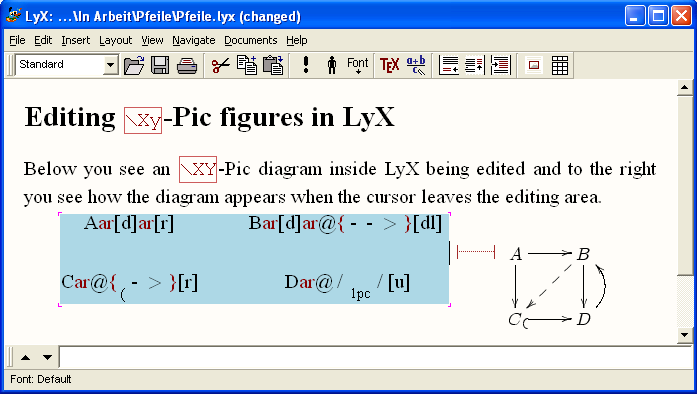In this note, we describe how XY -pic can be used from inside LY X, how diagrams can be created and edited. We have tested the following using LY X version 1.3.6-1 running under WindowsXP.

It is not our intention to write another introduction to XY -Pic, rather our motivation is to give an introduction how the most important commands work inside LY X, since the keystrokes as explained in the XY -Pic manual will not always function correctly inside LY X.

### 2 Preparation

The following requires that the LATEX-packages xypic and preview are installed in the LATEX system. They are available from CTAN, see at , resp. at . After freshly installig them, it may be necessary, to run Edit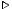Reconfigure from the main LY X menu. The steps to a first diagram output in LY X are then:

1. Activate and test preview:

1. Open LY X, choose EditPreferencesLook and FeelGraphics and place a check-mark at Instant Preview.
2. Test, if instant-preview works by opening a LY X-document and entering any math-formula, e.g. a + b = c.
3. Move the cursor out of the formula, and watch it change its appearance to look just like in the finished dvi- or postscript document.
2. Activate and test xypic:

1. Choose LayoutDocumentPreamble and type
\usepackage[all]{xy}.
2. Inside your LY X-Document, enter the text
\xymatrix{A \ar[r] & B} .
3. Select the whole text and choose InsertMathDisplay Formula, or use the corresponding keyboard shortcut C-M.
4. Move the mouse cursor out of the editing box and wait for a split second to see an arrow appear: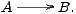### 3 Commutative diagrams

The following diagram, which is taken from the documentation of XY -Pic by its creator Kristoffer H. Rose, will provide an example for many of the features available with that package. Its source code is:

\xymatrix{
U     \ar@/{}_{1pc}/[ddr]_\psi\ar@/{}^{1pc}/[drr]^\varphi
\ar@{.>}[dr]|-{(x,y)}\\
& X \times_Z Y \ar[d]^q \ar[r]_p  &  X \ar[d]_f\\
& Y \ar[r]^g                      &  Z }

Again, to turn this code into a graphical output, select it all at once starting from the \xymatrix{ ... up to the closing brace ... } and turn it into display-math as explained above. A moment after the cursor leaves the math-area, you should see the diagram in its full graphical glory as shown below.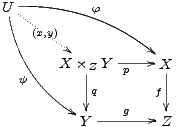#### 3.1 The matrix layout of diagrams

xymatrix uses a matrix to define the layout of the vertices of a diagram. For the above example, we need a 3 × 3-matrix of which 5 entries are used for the vertices U, X ×ZY , X, Y , Z, the other positions remaining empty. In this case, the following matrix determines the layout:

\xymatrix{
U                       \\
& X\times_Z Y   & X  \\
& Y             & Z    }

The pattern should be familiar from LATEX: We see three rows, each row being terminated by the end-of-line-marker \\ . Each line consists of entries, separated by the ampersand &.

#### 3.2 Arrows

Having entered the vertices, we add arrows between them. The basic xypic-command to produce an arrow is \ar , it is entered into the cell of the matrix where the arrow is to start. The target of the arrow is defined by direction commands u (up) d (down) l (left), or r (right). These can be combined to a path and enclosed in square brackets. As an example, the arrows from the vertex U in the upper left corner down and right to the vertices X ×ZY , Y , and X are, respectively, defined as \ar[dr], \ar[ddr] and \ar[drr]. Thus the above diagram with all arrows added becomes:

\xymatrix{
U \ar[ddr] \ar[drr] \ar[dr]\\
& X \times_Z Y \ar[d] \ar[r]& X \ar[d]\\
& Y \ar[r] & Z }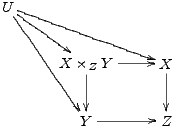#### 3.3 Labels

Labels are attached to arrows by affixing them as upper or lower indices to the \ar-command. Thus, \ar[drr]^\varphi defines an arrow going one cell down, two to the right and having the label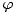attached above. To attach a label below the arrow, make it a lower index as in \ar[ddr]_\psi. This explanation is correct only for arrows pointing to the right. More precisely, imagine looking along the arrow in the direction it is pointing. Then an upper index places a label to the left and a lower index places it to the right. Consequently, an arrow pointing from right to left, such as \ar[l]^\alpha_\beta will have label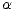below and label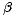above the arrow, i.e.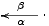Using the character | instead of ^ or _ , it is even possible to place the label right onto the arrow, obscuring part of its shaft.

Normally, a label is placed halfway between an arrow's start and target objects. In the first diagram, the central arrow starting in U has the label (x,y) in the middle of the arrow's shaft, rather than in the middle between the two objects it connects. This is achieved by prefixing the label with a minus sign, here: \ar[dr]|-{(x,y)}.

\xymatrix{
U \ar[ddr]_\psi \ar[drr]^\varphi\ar[dr]|-{(x,y)}\\
& X \times_Z Y \ar[d]^q \ar[r]_p& X \ar[d]_f\\
& Y \ar[r]^g & Z }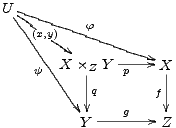#### 3.4 Arrow modification

Modification of the design, the form or the positioning of arrows are introduced by the @-character. This is followed by a pair of matching brackets, where the form of the bracket pair, { } or < > or / / indicates, whether we want to modify the design, the shift or the curvature of the arrow. Various modifactions can be applied to an arrow at the same time.

##### 3.4.1 Arrow design

Various designs such as solid, dotted, dashed or double are possible for the shaft of an arrow. These can be combined with various ends and various tips. In general, the design of an arrow is described by following the command \ar immediately by an @-sign and a pair of braces {...} containg characters describing the end, the shaft and the tip of the arrow. These characters are chosen to give some form of ASCII-rendering of the real thing. For instance \ar@{>..>>} produces an arow with split end, a dotted shaft and double head. A number of other arrow designs is given in the table below. Note that the ends of embedding arrows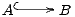are described by raising or lowering opening parentheses, such as in \ar@{^(->}[r].

 Result Source code in LY X\ar\ar@{-->}\ar@{..>}\ar@{~>}\ar@{->>}\ar@{-->>}\ar@{>->>}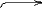\ar@{_(->}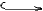\ar@{^(->}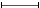\ar@{|-|}

Following the @-character by either a 2, 3, _,or a ^, we can produce arrows with double, triple shaft or arrows showing only the lower or upper half of their tips and ends. Arrows need not have tips nor ends, as the last example shows :

 Result Source code for LY X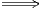\ar@2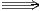\ar@3\ar@_{->}\ar@^{->}\ar@^{>>->>}\ar@{^<-_>}\ar@2{--}

#### 3.5 Arrow positioning

Arrows are shifted sideways with the modifier @<... > where the ellipsis is replaced by a positive or negative measure. For instance, to design a pair of mutually opposing arrows between two nodes, we shift them to see them apart. Note that the direction of the shift (positive) is to the left if one looks along the arrow. Thus

$\xymatrix{\circ \ar@<1ex>[r]& \circ \ar@<1ex>[l]}$

produces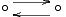##### 3.5.1 Inline or centered diagrams

Arrows and diagrams can be used inline, as this one: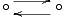. For this they should be placed inside an inline math-environment $...$ or $$...$$. To center a diagram put it inside display-math patrrentheses $and$. Diagrams constructed inline can later be centered, or, conversely, centered diagrams can be changed to inline formuls with EditMathAlignment.

#### 3.6 Bending arrows

There are two simple methods making arrows bend. The one is by giving an explicit value by which the midpoint of the arrow's shaft is raised or depressed, the other is by forcing the arrow to leave its origin in a prescribed compass direction and to make him enter the target at another direction. The necessary bending of the arrow is determined automatically. We describe both methods.

##### 3.6.1 Raising the shaft

For bending arrows we use the modifier @/.../ . The ellipsis stands for a TEX-measure which needs to be entered as a lower or upper index. Whereas in xypic, we could simply write, e.g. \ar@/_1pc/ for an arrow bending 1pc downwards, this cannot directly be done in LY X. It is necessary, to provide an empty object {}, relative to which the index is placed, and the measure must be enclosed in braces, such as e.g. \ar@/{}_{1pc}/. As an example, here are two opposing arrows between A and B, each bending by .5 pica, given by the following source code:

$\xymatrix{A \ar@/{}_{.5pc}/[r] & B \ar@/{}_{.5pc}/[l]}$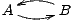##### 3.6.2 Specifying exit- and entrance directions.

An alternative for making arrows bend is by specifying their compass direction as they are leaving their source and their direction from which they enter their target. Instead of north, north-east, east, etc., the directions are named u, ur, r, dr, d, dl, l, ul, standing for up, up-right, right, down-right, etc.. A direction is specified as @(out,in) where out stands for the direction the first object is left and in stands for the direction from which the target is entered. As an example, we show some bending arrows and a loop, which arises when we do not specify a target for an arrow, just its incoming and outgoing direction: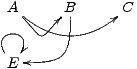$\xymatrix{A \ar@(dr,dl)[r]\ar@(dr,dl)[rr] &B\ar@(d,r)[dl] &C \\ E \ar@(ul,ur)}$

#### 3.7 Modifying vertices

The above example is reminiscent of an automata diagram, except that in such a diagram states would be shown inside small circles, with double circles denoting final states.

##### 3.7.1 Framing objects

With XY -Pic, entries can obtain a single or a double frame, such as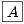or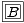by prefixing an entry with *[F-] or *[F=] and enclosing the portion of the entry to be framed in braces. Normally, the frame will be very tight so that it must be widened by prefixing with + or with ++. Round frames, such as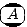and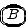are obtained by specifying the shape as [o]. So the latter figure was constructed as *++[o][F=]{B}. This way, the following automaton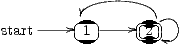can be typeset as

$\xymatrix{\txt{start}\ar[r] & *++[o][F]{1}\ar[r] & *++[o][F=]{2}\ar@(ur,dr)\ar@(ur,ul)[l] }$.

The LATEX command \entrymodifiers={...} will make a certain entrystyle the default, that can, of course be overridden for individual entries. Thus, after \entrymodifiers={++[o][F-]}, all following entries inside XY -matrices would be encircled.

### 4 Using LY X's math editor

As an alternative to writing the xypic code, then transforming it into a math-editing environment by marking it and applying C-m, or C-M, one may construct and modify the whole xypic-diagram inside LY X's math editor. We describe the editing steps for a figure just like the one above.

• Determine, which matrix-size you need in order to place all vertices into a square matrix. For the figure from section 1, for instance, a 3×3-matrix is required.
• With C-m or C-M open a formula environment and enter: \xymatrix. This produces a 1×1-matrix.
• Additional rows can be entered with C-Enter and new colums with EditMathAdd Column, resp. M-m c i.
• Type the nodes into the correct positions of the matrix. If you move the cursor out of the matrix, you should see a first rendering of the node layout.
• Next add the arrows at the nodes where they should emanate by typing \ar[p], where p can be any path made up from the characters u, d, l, r. Make sure that the path indeed leads to a node available within the matrix. Otherwise, the figure will not display as the cursor leaves the editing area.
• Next, label the arrows by attaching upper or lower indices to their right end.
• Finally, modify the appearance of the arrows by entering @-modifiers @{...}, @<...>, @(...,...) or @/.../. Two are two caveats:
• A pair of braces {...} inside a formula must be entered by typing just  \{  without the closing brace. It will be supplied automatically by the math editor.
• The code for bending arrows, which in xypic is @/_dim/ or @/^dim/ where dim is the dimension, must be entered by attaching dim as upper or lower index to an empty object pair of braces. The keystrokes inside the math editor, therefore, are either @/\{_dim or @/\{^dim .

It is often convenient to stretch the horizontal or vertical dimension of a digram by using sacing commands for rows and columms. E.g. \xymatrix@R=1pc{...} defines an XY-matrix with row spacing of 1 pica. Unfortunately, this does currently not work inside LY X, since the @-character is interpreted by LY X as ending the XY-matrix-macro.

### References

   Kristoffer H. Rose: XY -Pic User's Guide. Version 3.7, Feb. 16, 1999. Available as part of the xypic LATEX package.